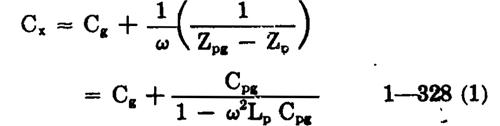Miller Oscillator with Cricuit

1428. The Miller oscillator is the crystal equivalent of a Hartley oscillator in which no mutual inductance exists between the plate-to cathode and gridt o.cithode inductances. ( See figure 1-140.) The Miller oscillator has an average frequency deviation of approximately 1.5 times that of the Pierce circuit. The plate circuit must appear inductive in order that the correct phase shift will be produced in E, the plate r-f voltage, to compensate for the

resistance in the feed-back arm, since this resist ance prevents the necessary 180.-degree phse rotation of the equivalent generator voltage of the amplifier from occurring entirely in the feedback circuit. The effective load capacitance into which the crystal unit operates is, approximately,• —
where Z1)g and Z, are both considered as unsigned magnitudes, and the various symbols correspond to those in figure 1-140. Since the load capacitance is a function of the frequency, a Miller oscillator cannot be operated at more than one frequency and still present the same load capacitance to each crystal unit except by providing for an adjustment of the circuit parameters. In spite of its greater frequency instability and lack of circuit simplicity as compared with the Pierce circuit, the Miller design is the one most widely used in crystal oscill ators. The reason for this popularity is the greater output that can be obtained for the same crystal drive level. En either the Pierce or the Miller basic circuit, the output cnnot exceed the voltage across Zpg, the largest single impedance in the plate tank circuit. In the Pierce circuit, the maximum voltage is thus the maximum permissible across the crystal unit; in the Miller circuit the maximum voltage is (k + 1) times the maximum permissible vo1tige across the crystal unit, where k is the gain of the stage, equal to Ep/Eg. This gain, theoretically (not practically), can approach the mu of the tube as a limit when the load impedance, ZL, is large comp ared with R. Thus, the use of a Miller circuit permits a saving of one amplifier stage.
1-329. The feed-back capacitance of the Miller circ uit is, normally, simply the plate-to-grid inter- electrode capacitance of the tube. It cannot, of course, be less than this unless an inductive shunt is connected between the plate and grid. When a pentode is used, it is usually necessary to insert a small feed-back capacitance on the order of a few micromicrofarads. The waveform in the output is improved by the use of a tuned tank circuit having a low L/C ratio in place of L. The plate tank must be tuned to a frequency above the oscillator freq uency, in order that the tank impedance will app ear inductive. Such an arrangement also ensures a large effective L of high Q. A vgiriable capaci tance in the plate tank facilitates adjustments to obtain the correct load capacitance for the crystal unit.